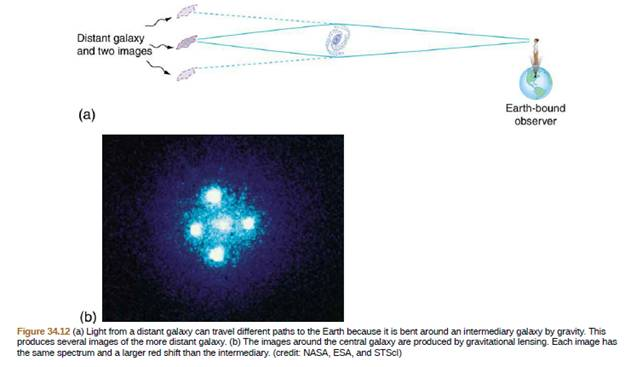Chapter 34, Problem 14CQ

Chapter
Section
Textbook Problem

Suppose you measure the red shifts of all the images produced by gravitational lensing, such as in Figure 34.12. You find that the central image has a red shill less than the outer images, and those all have the same red shift. Discuss how this not only shows that the images are of the same object, but also implies than the red shift is not affected by taking different paths through space. Does it imply that cosmological red shifts are not caused by traveling through space (light getting tired, perhaps)?To determine

The phenomenon which shows that for figure 1, the images are of the same object as well as the red shift is not affected by taking different paths through space. Also, state whether the given figure will imply that that cosmological red shifts are not caused by traveling through space or not.

Explanation

The given figure is shown below.

Figure 1

The redshift causes release of light of a larger wavelength from a distant star as compared to a nearby star. This is the scenario when Universe is assumed to be homogenous. But, alterations in light are caused by the composition of universe...

Still sussing out bartleby?

Check out a sample textbook solution.

See a sample solution

The Solution to Your Study Problems

Bartleby provides explanations to thousands of textbook problems written by our experts, many with advanced degrees!

Get Started

Find more solutions based on key concepts